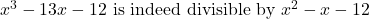## Use the Factor Theorem to prove x^3 – 13x – 12 is divisible by x^2 – X – 12

Question

Use the Factor Theorem to prove x^3 – 13x – 12 is divisible by x^2 – X – 12

in progress 0
7 months 2021-07-20T21:30:35+00:00 1 Answers 8 views 0

See Below.

Step-by-step explanation:

We want to prove that: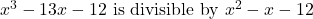We can factor the divisor: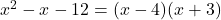According to the Factor Theorem, if we have a polynomial P(x) divided by a binomial in the form of (xa) and if P(a) = 0, then the binomial is a factor of P(x).

Our two binomial factors our (x – 4) and (x + 3). Thus, a = 4 and a = -3.

Evaluate the polynomial for both of these factors: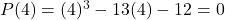And: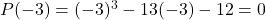Since both yielded zero, the original polynomial is divisible by both (x – 4) and (x + 3) or x² – x – 12. Hence: